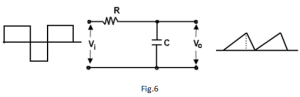# Differentiating Circuit and Integrating Circuit

## Differentiating Circuit

A circuit in which output voltage is directly proportional to the derivative of the input  is known as a differentiating circuit.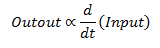A differentiating circuit is a simple series RC circuit where the output is taken across the resistor R.

The circuit is suitably designed so that the output is proportional to the derivative of the input.

Thus if a d.c. or constant input is applied to such a circuit, the output will be zero.Fig.1 shows a typical differentiating circuit.

In order to achieve good differentiation, the following two conditions should be satisfied:

1. The time constant RC of the circuit should be much smaller than the time period of the input wave.
2. The value of XC should be 10 or more times larger than R at the operating frequency.

Fulfilled these conditions, the output across R will be the derivative of the input.

Let ei be the input alternating voltage

And let  i be the resulting alternating current.

The charge q on the capacitor  C at any instant is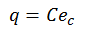Now,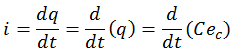Or,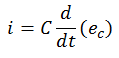Since the capacitive reactance is very much larger than R, the input voltage can be considered equal to the capacitor voltage with negligible error i.e.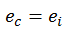So,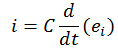Output voltage,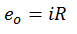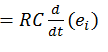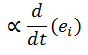HenceOutput Waveforms:

The output waveform from a differentiating circuit depends upon the time constant and shape of the input wave. Three important cases will be discussed here.

1. When input is a square wave:

When the input fed to a differentiating circuit is a square wave, output will consist of sharp narrow pulses as shown in Fig.2.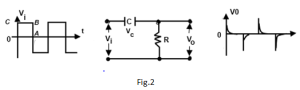During the OC part of input wave, its amplitude changes abruptly and hence the differentiated wave will be a sharp narrow pulse as shown in fig.2.

However, during the constant part CB of the input, the output will be zero because the derivative of a constant is zero.

Let us look at the physical explanation of this behavior of the circuit.

Since time constant RC of the circuit is very small w.r.t. time period of input wave and XC>>R,

The capacitor will become fully charged during the early part of each half-cycle of the input wave.

During the remainder part of the half-cycle, the output of the circuit will be zero because the capacitor voltage (ec) neutralizes the input voltage and there can be no current flow through R.

Thus we shall get sharp pulse at the output during the start of each half-cycle of input wave while for the remainder part of the half-cycle of input wave, the output will be zero.

Such pulses are used in many ways in electronics circuits e.g. in television transmitters and receivers, in multivibrators to initiate action etc.

2.     When input is a triangular wave:

When the input fed to a differentiating circuit is a triangular wave, the output will be a rectangular wave as shown in fig.3.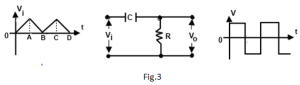During the period OA of the input wave, its amplitude changes at a constant rate and, therefore, the differentiated waveform has a constant value for the constant rate of change.

During the period AB of the input wave, the amplitude changes at a negative constant rate and, therefore, the differentiated wave has a negative constant value for the negative constant rate of change.

Thus when a triangular wave is fed to a differentiator, the output consists of a succession of rectangular waves of equal or unequal duration depending upon the shape of the input wave.

3.   When input is a sine wave:

When the input fed to the input of a differentiating circuit is a sine wave, the output will be a cosine wave. And a cosine wave input becomes an inverted sine wave at the output.

## Integrating Circuit

A circuit in which output voltage is directly proportional to the integral of the input, is known as an integrating circuit.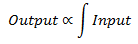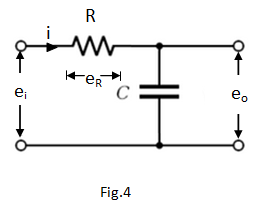In order to achieve good integration, the following two conditions must be fulfilled:An integrating circuit is a simple RC circuit with output taken across the capacitor C as shown in fig.4.

• The time constant RC o the circuit should be very large as compared to the time period of the input wave.
• The value of R should be 10 or more times larger than XC.

Let ei be the input alternating voltage and let i be the resulting alternating current.

Since R is very large as compared to capacitive reactance XC of the capacitor, it is assumed that voltage across R (i.e. eR) is equal to the input voltage i.e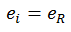Now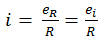The charge q on the capacitor at any instant is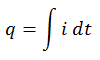Output voltage,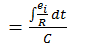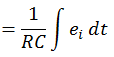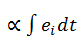Hence,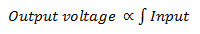Output Waveforms:

The output waveform from an integrating circuit depends upon time constant and shape of the input wave. Two important cases will be discussed here:

1.   When input is a square wave:

When the input fed to an integrating circuit is a square wave, the output will be a triangular wave as shown in fig.5.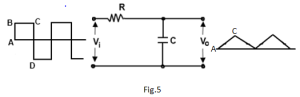As integration means summation, therefore, output from an integration circuit will be sum of all the input waves at any instant.

This sum is zero at A and goes on increasing till it becomes maximum at C.

After this, the summation goes on decreasing to the onset of negative movement CD of the input.

2.      When input is rectangular wave:

When the input fed to an integrating circuit is a rectangular wave, the output will be a triangular wave as shown in fig.6.